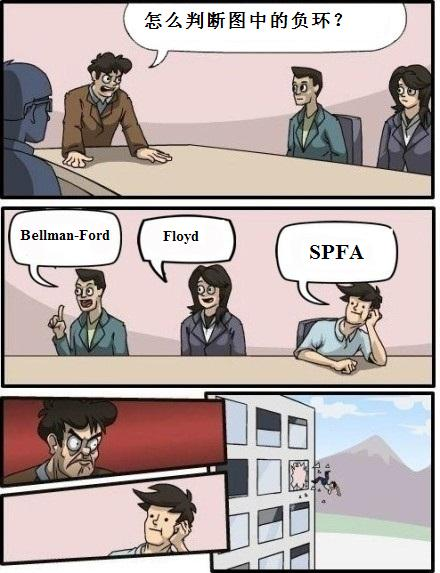# 博客

## UOJ Round #2 题解

2014-12-06 22:18:23 By vfleaking

### 跳蚤公路

#### 算法四\begin{equation} \min \{ kx + f[n][v][k] \} \geq \min \{ jx + f[n - 1][v][j] \} \end{equation}

\begin{equation} kx + f[n][v][k] \geq \min \\{ jx + f[n - 1][v][j] \\} \end{equation}

\begin{equation} kx + f[n][v][k] \geq jx + f[n - 1][v][j] \end{equation}

#### 其他算法

（可能有人会问为何要建一个超级源，用强连通分量中任意一个点不行么？当然不行。比如某个点走到负环上需要走 $10^9$ 的距离，但是负环的权值仅为 $-1$。于是就得很久很久之后才会让这个点到自己的最短距离比 $0$ 小。感觉这算法八九不离十了，所以给的大约 80 分。）

UPD：（上面这个算法是错的……T_T……大家还是写算法四吧，质量有保障）

### 树上GCD

#### 算法四

1. $u,v$ 均在 $c$ 的子树内，此时 LCA 即为 $c$。和算法三类似，只是第 $i$ 条链中高度为 $d$ 的倍数的点的数量需要在处理出 $cnt$ 数组后花 $h_i/d$ 的时间统计，所以复杂度是$O(n \log n)$。
2. $u$ 在 $c$ 的子树内，而 $v$ 不在。我们把当前树的根节点记为 $root$，$father[c]$ 到 $root$ 的路径为 $father[c]=a_1,a_2,\cdots,a_{k-1},a_k=root$。记 $c$ 下面的子树高度为 $H$，$a_i$ 旁边伸出的子树（即不包含 $a_{i-1}$ 的)的高度为 $h_i$。枚举 $a_i=LCA(u,v)$，那么 $v$ 位于 $a_i$ 旁边的子树中，然后我们仍然枚举 $d$，用 $h_i/d$ 的时间求出 $a_i$ 子树中高度为 $d$ 的倍数的结点数量；但我们还需要知道 $c$ 的子树中有多少点相对于 $a_i$ 的高度是 $d$ 的倍数，也就是说我们要在 $c$ 子树的 $cnt$ 数组中查询下标间隔为 $d$ 的子序列中的元素之和。注意到间隔为 $d$ 时，至多只有 $d$ 种这样的子序列，我们对重复查询进行记忆化。于是对于 $d<\sqrt{H}$，查询的复杂度不超过 $d\cdot H/d=H$，总共是 $\sqrt{H}\cdot H$；对于 $d>\sqrt{H}$，单次查询的复杂度为 $H/d<\sqrt{H}$。

## 评论

saffah

delayyy

Picks

wyfcyx
Sofa~~~
zhangzj

thomount

zangfenziang

Gromah
Orz
Trinkle

dmcyer
@
wyfcyx
@vfleaking 为什么spfa被搞出去？
liu_cheng_ao

lyx_cjz
T3启发式合并的复杂度似乎也是$O \left ( n \sqrt{n} \right )$？
lyx_cjz

djq_cpp
T3点分FFT能不能O(n polylog(n))啊。。 重心将整棵树划分为若干个部分 对于同一部分内的u, v，递归处理 对于不同部分内的u, v 如果1与重心的连线经过u（或v）所在的子树 那么枚举u（或v）和1点分树上的lca，分别fft 如果不经过，直接fft （可能是假的 （log数量好多啊。。。tle预定
boshi

Ameiyo

C3H5ClO

feecle6418
cf 上出了 t1 题意一样的题，只不过是最小化操作次数：https://codeforces.com/contest/1685/problem/C，可以证明至多两次操作即可